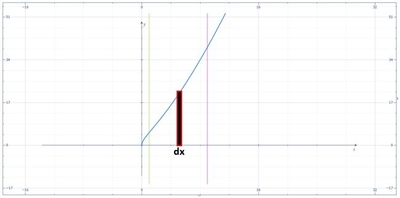# Find the total area of the region between the curve and the x-axis. y = (x + 4)\sqrt x; 1 \leq...

## Question:

Find the total area of the region between the curve and the x-axis.

{eq}y = (x + 4)\sqrt x; 1 \leq x \leq 9 {/eq}

a) 761

b) {eq}\frac{328}{3} {/eq}

c) 346

d) {eq}\frac{2492}{15} {/eq}

## Area of the Region:

To compute the area of the region bounded by the curve and line we can use the vertical strip method with its formula {eq}\displaystyle A=\int_{a}^{b}ydx {/eq} where {eq}y {/eq} and {eq}dx {/eq} are the length and width of the rectangular strip.

Below is the graph,The area of the region is,

{eq}\displaystyle A=\int_{1}^{9}ydx {/eq}

{eq}\displaystyle A=\int_{1}^{9}\left [ \left ( x+4 \right )\sqrt{x} \right ]dx {/eq}

Integrate,

{eq}\displaystyle A=\left [ \frac{2}{5}x^{\frac{5}{2}}+\frac{8}{3}x^{\frac{3}{2}} \right ]^{9}_{1} {/eq}

{eq}A=\frac{46}{15} {/eq}

{eq}A=\:3.06667 {/eq}